# Variational Autoencoder with Tensorflow – VII – KL loss via model.add_loss()

I continue my series on options regarding the treatment of the Kullback-Leibler divergence as a loss [KL loss] in Variational Autoencoder [VAE] setups.

Our objective is to find solutions which avoid potential problems with the eager execution mode of present Tensorflow 2 implementations. Popular recipes of some teaching books on ML may lead to non-working codes in present TF2 environments. We have already looked at two working alternatives.

In the last post we transferred the “mu” and “log_var” tensors from the Encoder to the Decoder and fed some Keras standard loss functions with these tensors. These functions could in turn be inserted into the model.compile() statement. The approach was a bit complex because it involved multi-input-output model definitions for the Encoder and Decoder.

The present article will discuss a third and lighter approach – namely using the Keras add_loss() mechanism on the level of a Keras model, i.e. model.add_loss().

The advantage of this function is that its parameter interface is not reduced to the form of standardized Keras cost function interfaces which I used in my last post. This gives us flexibility. A solution based on model.add_loss() is also easy to understand and realize on the programming level. It is, however, an approach which may under certain conditions reduce performance by roughly a factor between 1.3 and 1.5 – which is significant. I admit that I have not yet understood what the reasons are. But the concrete solution version I present below works well.

# The strategy

The way how to use Keras’ add_loss() functionality is described in the Keras documentation. I quote from this part of TF2’s documentation about the use of add_loss():

This method can also be called directly on a Functional Model during construction. In this case, any loss Tensors passed to this Model must be symbolic and be able to be traced back to the model’s Inputs. These losses become part of the model’s topology and are tracked in get_config.

The documentation also contains a simple example. The strategy is to first define a full VAE model with standard mu and log_var layers in the Encoder part – and afterwards add the KL-loss to this model. This is depicted in the following graphics: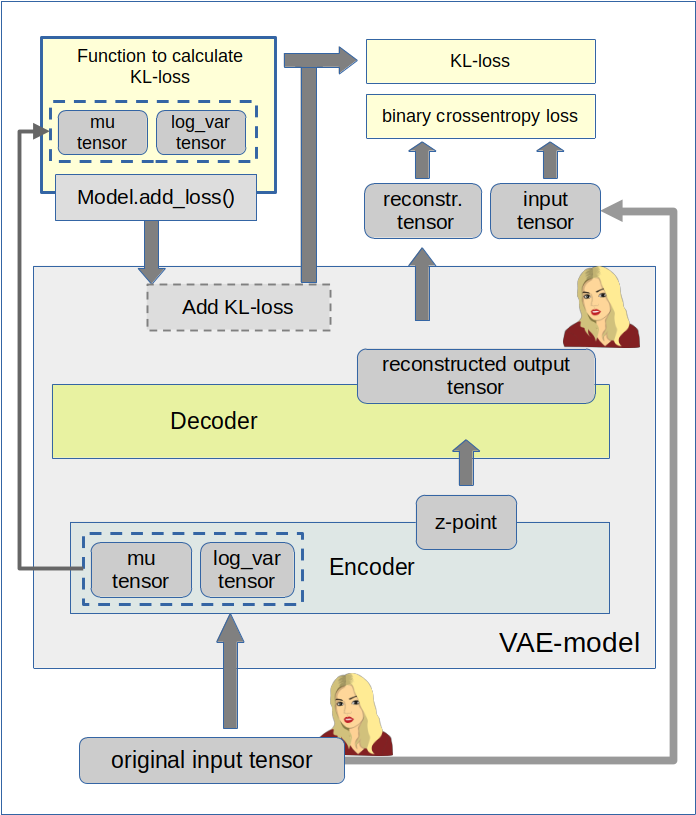We implement this strategy below via the Python class for a VAE setup which we have used already in the last 4 posts of this series. We control the Keras model setup and the layer construction by the parameter “solution_type”, which I have introduced in my last post.

# Cosmetic changes to the Encoder/Decoder parts and the model creation

The class method _build_enc(self, …) can remain as it was defined in the last post. We just have to change the condition for the layer setup as follows:

Change to _build_enc(self, …)

```... # see other posts
...
# The Encoder Model
# ~~~~~~~~~~~~~~~~~~~
# With extra KL layer or with vae.add_loss()
if solution_type == 0 or solution_type == 2:
self.encoder = Model(self._encoder_input, self._encoder_output)

# Transfer solution => Multiple outputs
if solution_type == 1:
self.encoder = Model(inputs=self._encoder_input, outputs=[self._encoder_output, self.mu, self.log_var], name="encoder")
```

Something similar holds for the Decoder part _build_decoder(…):

Change to _build_dec(self, …)

```... # see other posts
...
# The Decoder model
# solution_type == 0/2: Just the decoded input
if self.solution_type == 0 or self.solution_type == 2:
self.decoder = Model(self._decoder_inp_z, self._decoder_output)

# solution_type == 1: The decoded tensor plus the transferred tensors mu and log_var a for the variational distribution
if self.solution_type == 1:
self.decoder = Model([self._decoder_inp_z, self._dec_inp_mu, self._dec_inp_var_log],
[self._decoder_output, self._dec_mu, self._dec_var_log], name="decoder")
```

A similar change is done regarding the model definition in the method _build_VAE(self):

Change to _build_VAE(self)

```        solution_type = self.solution_type

if solution_type == 0 or solution_type == 2:
model_input  = self._encoder_input
model_output = self.decoder(self._encoder_output)
self.model = Model(model_input, model_output, name="vae")

... # see other posts
...
```

# Changes to the method compile_myVAE(self, learning_rate)

More interesting is a function which we add inside the method compile_myVAE(self, learning_rate, …).

Changes to compile_myVAE(self, learning_rate):

```    # Function to compile the full VAE
# ~~~~~~~~~~~~~~~~~~~~~~~~~~~~~~~~~~~~~~~~~~~~~
def compile_myVAE(self, learning_rate):

# Optimizer
# ~~~~~~~~~
# save the learning rate for possible intermediate output to files
self.learning_rate = learning_rate

# Parameter "fact" will be used by the cost functions defined below to scale the KL loss relative to the BCE loss
fact = self.fact

# Function for solution_type == 1
# ~~~~~~~~~~~~~~~~~~~~~~~~~~~~~~~~~
@tf.function
def mu_loss(y_true, y_pred):
loss_mux = fact * tf.reduce_mean(tf.square(y_pred))
return loss_mux

@tf.function
def logvar_loss(y_true, y_pred):
loss_varx = -fact * tf.reduce_mean(1 + y_pred - tf.exp(y_pred))
return loss_varx

# Function for solution_type == 2
# ~~~~~~~~~~~~~~~~~~~~~~~~~~~~~~~~
# We follow an approach described at
# https://www.tensorflow.org/api_docs/python/tf/keras/layers/Layer
# NOTE: We can NOT use @tf.function here
def get_kl_loss(mu, log_var):
kl_loss = -fact * tf.reduce_mean(1 + log_var - tf.square(mu) - tf.exp(log_var))
return kl_loss

# Required operations for solution_type==2 => model.add_loss()
# ~~~~~~~~~~~~~~~~~~~~~~~~~~~~~~~~~~~~~~~~~
res_kl = get_kl_loss(mu=self.mu, log_var=self.log_var)
if self.solution_type == 2:

# Model compilation
# ~~~~~~~~~~~~~~~~~~~~
if self.solution_type == 0 or self.solution_type == 2:
self.model.compile(optimizer=optimizer, loss="binary_crossentropy",
metrics=[tf.keras.metrics.BinaryCrossentropy(name='bce')])

if self.solution_type == 1:
self.model.compile(optimizer=optimizer
, loss={'vae_out_main':'binary_crossentropy', 'vae_out_mu':mu_loss, 'vae_out_var':logvar_loss}
#, metrics={'vae_out_main':tf.keras.metrics.BinaryCrossentropy(name='bce'), 'vae_out_mu':mu_loss, 'vae_out_var': logvar_loss }
)
```

I have supplemented function get_kl_loss(mu, log_var). We explicitly provide the tensors “self.mu” and “self.log_var” via the function’s interface and thus follow one of our basic rules for the Keras add_loss()-functionality (see post IV).
Note that this is a MUST to get a working solution for eager execution mode!

Interestingly, the flexibility of model.add_loss() has a price, too. We can NOT use a @tf.function indicator here – in contrast to the standard cost functions which we used in the last post.

Note also that I have added some metrics to get detailed information about the size of the crossentropy-loss and the KL-loss during training!

# Cosmetic change to the method for training

Eventually we must include solution_type==2 in method train_myVAE(self, x_train, batch_size, …)

Changes to train_myVAE(self, x_train, batch_size,…)

```... # see other posts
...
if self.solution_type == 0 or self.solution_type == 2:
self.model.fit(
x_train
, x_train
, batch_size = batch_size
, shuffle = True
, epochs = epochs
, initial_epoch = initial_epoch
)

if self.solution_type == 1:
self.model.fit(
x_train
#               Working
#                , [x_train, t_mu, t_logvar] # we provide some dummy tensors here
# by dict:
, {'vae_out_main': x_train, 'vae_out_mu': t_mu, 'vae_out_var':t_logvar}
, batch_size = batch_size
, shuffle = True
, epochs = epochs
, initial_epoch = initial_epoch
#, verbose=1
, callbacks=[MyPrinterCallback()]
)

```

# Some results

We can use a slightly adapted version of the Jupyter notebook cells discussed in post V

Cell 6:

```from my_AE_code.models.MyVAE_2 import MyVariationalAutoencoder

z_dim         = 12
solution_type = 2
fact          = 6.5e-4

vae = MyVariationalAutoencoder(
input_dim = (28,28,1)
, encoder_conv_filters = [32,64,128]
, encoder_conv_kernel_size = [3,3,3]
, encoder_conv_strides = [1,2,2]
, decoder_conv_t_filters = [64,32,1]
, decoder_conv_t_kernel_size = [3,3,3]
, decoder_conv_t_strides = [2,2,1]
, z_dim = z_dim
, solution_type = solution_type
, act   = 0
, fact  = fact
)

```

Cell 11:

```BATCH_SIZE = 256
EPOCHS = 37
PRINT_EVERY_N_BATCHES = 100
INITIAL_EPOCH = 0

if solution_type == 2:
vae.train_myVAE(
x_train[0:60000]
, batch_size = BATCH_SIZE
, epochs = EPOCHS
, initial_epoch = INITIAL_EPOCH
)

```

Note that I have changed the BATCH_SIZE to 256 this time; the performance got a bit better then on my old Nvidia 960 GTX:

```Epoch 3/37
235/235 [==============================] - 10s 44ms/step - loss: 0.1135 - bce: 0.1091 - kl: 0.0044
Epoch 4/37
235/235 [==============================] - 10s 44ms/step - loss: 0.1114 - bce: 0.1070 - kl: 0.0044
Epoch 5/37
235/235 [==============================] - 10s 44ms/step - loss: 0.1098 - bce: 0.1055 - kl: 0.0044
Epoch 6/37
235/235 [==============================] - 10s 43ms/step - loss: 0.1085 - bce: 0.1041 - kl: 0.0044
```

This is comparable to data we got for our previous solution approaches. But see an additional section on performance below.

# Some results

As in the last posts I show some results for the MNIST data without many comments. The first plot proves the reconstruction abilities of the VAE for a dimension z-dim=12 of the latent space.

MNIST with z-dim=12 and fact=6.5e-4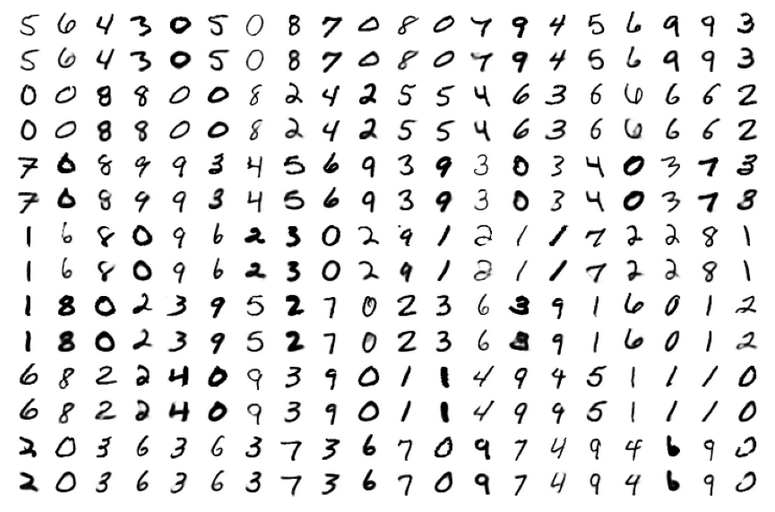For z_dim=2 we get a reasonable data point distribution in the latent space due to the KL loss, but the reconstruction ability suffers, of course:

MNIST with z-dim=2 and fact=6.5e-4 – train data distribution in the z-space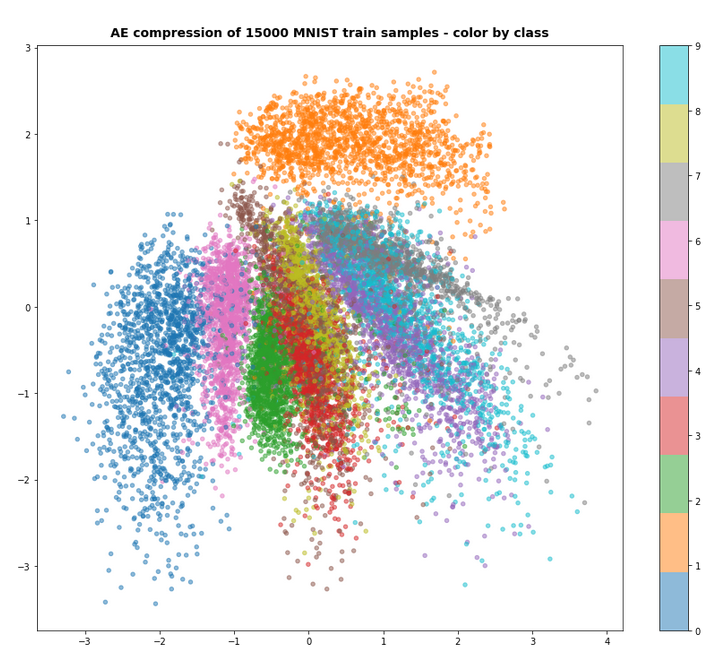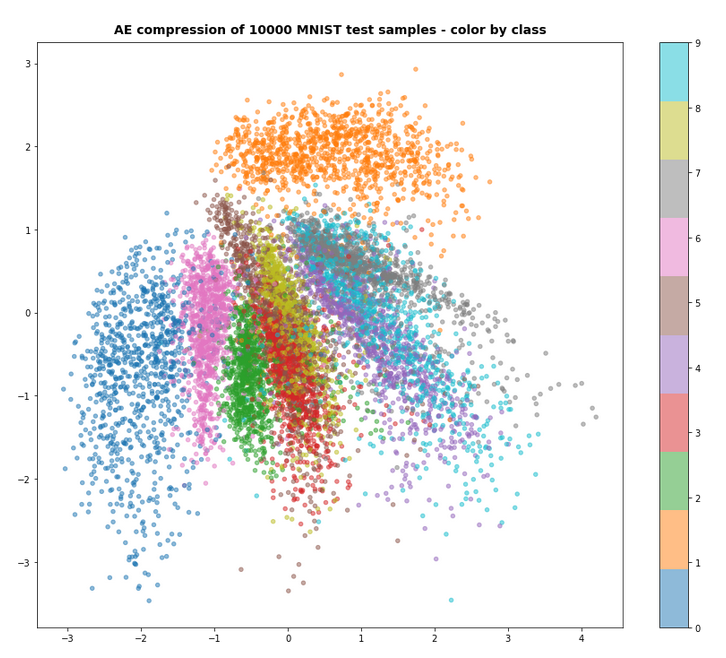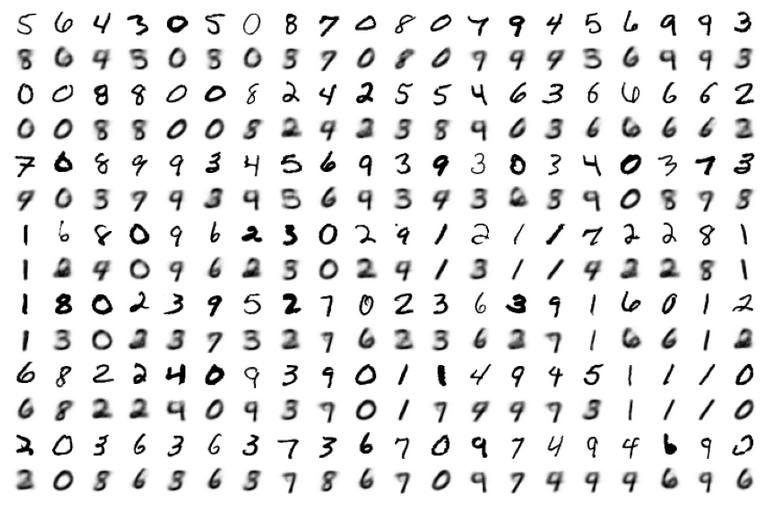For a dimension of z_dim=2 of the latent space and MNIST data we get the following reconstruction chart for data points in a region around the latent space’s origin

# A strange performance problem when no class is used

I also tested a version of the approach with model.add_loss() without encapsulating everything in a class. But with the same definition of the Encoder, the Decoder, the model, etc. But all variables as e.g. mu, log_var were directly kept as data of and in the Jupyter notebook. Then a call

```n_epochs      = 3
batch_size    = 128
initial_epoch = 0
vae.fit( x_train[0:60000],
x_train[0:60000],   # entscheidend !
batch_size=batch_size,
shuffle=True,
epochs = n_epochs,
initial_epoch = initial_epoch
)
```

reduced the performance by a factor of 1.5. I have experimented quite a while. But I have no clue at the moment why this happens and how the effect can be avoided. I assume some strange data handling or data transfer between the Jupyter notebook and the graphics card. I can provide details if some developer is interested.

But as one should encapsulate functionality in classes anyway I have not put efforts in a detail analysis.

# Conclusion

In this article we have studied an approach to handle the Kullback-Leibler loss via the model.add_loss() functionality of Keras. We supplemented our growing class for a VAE with respective methods. All in all the approach is almost more convenient as the solution based on a special layer and layer.add_loss(); see post V.

However, there seems to exist some strange performance problem when you avoid a reasonable encapsulation in a class and do the modell setup directly in Jupyter cells and for Jupyter variables.

In the next post
Variational Autoencoder with Tensorflow – VIII – TF 2 GradientTape(), KL loss and metrics
I shall have a look at the solution approach recommended by F. Chollet.